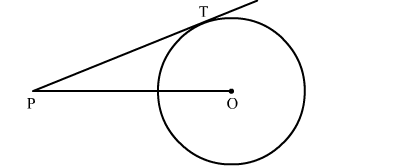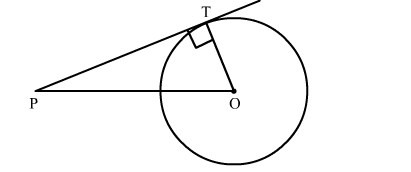# In the given figure, point P is 26 cm away from the centre O of a circle and the length PT of the tangent drawn from P to the circle is 24 cm.`
Question:

In the given figure, point P is 26 cm away from the centre O of a circle and the length PT of the tangent drawn from P to the circle is 24 cm. Then the radius of the circle is

(a) 10 cm
(b) 12 cm
(c) 13 cm
(d) 15 cmSolution:

Construction: Join OTWe know that the radius and tangent are perperpendular at their point of contact
In right triangle PTO
By using Pythagoras theorem, we have
PO2 = OT2 + TP2
⇒ 262 = OT2 + 242
676 = OT2 + 576
TP2 = 100
⇒ TP = 10 cm
Hence, the correct answer is option (a).